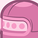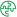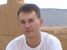App Development

Announcements
QlikWorld 2022, LIVE in Denver CO., May 16-19, 2022. REGISTER NOW TO RECEIVE EARLY BIRD PRICING
cancel
Showing results for
Did you mean:Contributor III

How to Use a Measure Result As Dimension?

Hello Everyone!

I was wondering if I can use a measure result condition as a Dimension (i.e. assuming I have measure X which is the avg time, I'd like to have a dimension like the following: X < 20, X=20 , X>20)

1 Solution

Accepted SolutionsMVP

A measure is calculated over dimension values. If used without dimensions then a measure returns only one value.

So if you want to use a measure as a dimension you probably want to use the measure values calculated for each of the dimension values of a dimension. So you need to use the aggr function to build a virtual table with the list of measures per dimension value.

Something like this:

Aggr(

If( avg(Time) < 20, 'X<20',

If( avg(Time)=20,'X=20','X>20'))

DimensionA, DimensionB, ...etc )

talk is cheap, supply exceeds demandMVP

A measure is calculated over dimension values. If used without dimensions then a measure returns only one value.

So if you want to use a measure as a dimension you probably want to use the measure values calculated for each of the dimension values of a dimension. So you need to use the aggr function to build a virtual table with the list of measures per dimension value.

Something like this:

Aggr(

If( avg(Time) < 20, 'X<20',

If( avg(Time)=20,'X=20','X>20'))

DimensionA, DimensionB, ...etc )

talk is cheap, supply exceeds demandTags
Community Browser